اولين سايت تخصصي آموزش و نگهداري و تعميرات ديگ بخار، ديگ آبگرم، ديگ روغن داغ و آموزش نگهداري و طراحي تاسيسات در ايران به صورت کاملا فارسي، و با استناد به آخرين دستاوردهي تکنولوژي ديگ هاي بخار و تاسيسات مربوطه، در جهان.:::| درج آگهي | فروشگاه | | درباره ما | تماس با ما |:::

HEAT TRANSFER IN THE RADIANT SECTION OF PETROLEUM HEATERS (part2): 1 - 2 - 3 - 4

EVALUATION OF THE EQUIVALENT SURFACE, aAcp

Acp is the area of a continuous plane replacing the row of tubes and may be taken as the product of the exposed tube length, and center to center distance between tubes, and the number of tubes in the exposed radiant row. a is the ratio of reception by the actual surface to reception by a continuous plane. Then the term aAcp is the tube area expressed as equivalent cold plane surface, i.e., the area of a plane which will absorb the same as the actual cold surface in the furnace.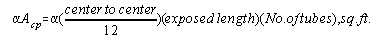Hottel8 gives a as a function of the ratio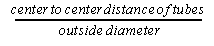as in Figure 5.

 The following example illustrates the method of calculating aAcp. Assume a radiant section of the following characteristics : Size of tubes 5" outside diameter Center to center distance of tubes 10" Exposed length of tube 30 ft. Total number of tubes 60 Arrangement of tubes, 2 rows on equilateral triangular spacing Number of tubes per row 30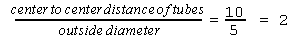a=0.984, i.e., total to 2 rows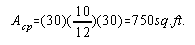aAcp=0.984(750)=738 sq.ft. equivalent cold plane surface.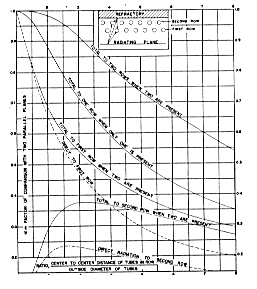Fig. 5 Distribution of Heat to One or Two Rows of Tubes Mounted on Refractory Wall and Irradiated from One Side.

Tubes on equalateral triangular centers; ordinate expressed on basis of heat transfered from a plane to a plane replacing tubes, or to infinite number of rows of tubes. These curves are a good approximation for tubes placed on rectangular or square centers.

EVALUATION OF THE FLAME EMISSIVITY, Pf

By definition, the emissivity of the flame is the ratio of the heat actually transmitted from the flame to the cold surface to the heat which would have been transmitted had the flame and the cold surface been perfect radiators. An illustrative example of this calculation is available in the literature.11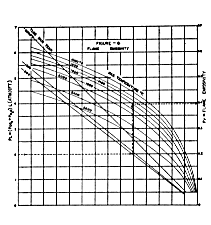Figure No. 6 gives Pf in terms of (PCO2+PH2O)L, tg, and ts for cracked gas fuel and a tube emissivity of 0.90. This plot is also a good approximation for fuel oil. The radiation cjharts of Hottel9 which were used in this calculation are included in the Appendix as Figures Nos. 13 and 14.

 PCO2 = partial pressure of carbon dioxide in the flue gas, atmospheres PH2O = partial pressure of water vapor in the flue gas, atmospheres L = mean length of radiant beam in the combustion chamber, feet PL = atmospheres-feet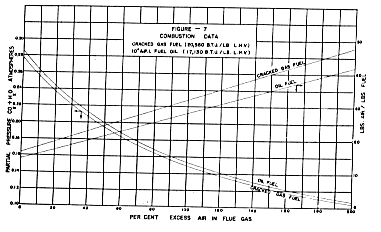Values of PCO2 + PH2O and the air-fuel ratio for typical cracked gas and oil fuels have been plotted on Figure No. 7. The analysis of the fuels on which these calculations are based are indicated in the Appendix.

The mean length, L, of the radiant beam in the combustion chamber may be estimated from Table I.

 Table I.-Mean Length of Radiant Beams in Various Gas Shapes12 From the values given by Hottel12 for the mean length, L, of radiant beams in various gas shapes, the following approximate values have been derived for use in practical oil heater design. These values may be used until more exact methods are available. Dimensional Ratio (length, width, height L in any order) Rectangular Furnaces 1. 1-1-1 to 1-1-3 1-2-1 to 1-2-4 2/3(Furnace Volume)1/3 2. 1-1-4 to 1-1-inf 1 x smallest dimension 3. 1-2-5 to 1-2-8 1.3 x smallest dimension 4. 1-3-3 to 1-inf-inf 1.8 x smallest dimension Cylindrical Furnaces 5. d x d 2/3 x diameter 6. d x 2d to d x inf 1 x diameter

EVALUATION OF THE OVERALL ECHANGE FACTOR, f

 Let At = total area of furnace surfaces in the radiant section= Acp + refractory surfaces unprotected by tubes, sq. ft aAcp = equivalent cold plane surface, sq.ft. Ar = effective refractory surface, sq.ft. (Ar = At - aAcp) Pc = emissivity of the ultimate heat-receiving surface, assumed =0.90 Pf = emissivity of the flame. Frc = fraction of all the radiation emitted from all the refractory in all directions, which, if not absorbed by the gas, would hit cold surface aAcp

The overall exchange factor, f, as defined by Hottel9, is then :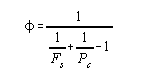(18)

Where(19)

Af = Area of the flame bundle, sq. ft.
In commercial furnaces Af may be considered equal to At, and equation (19) may be simplified to the form used in this study :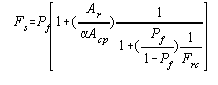(20)

The exact evaluation10 of Frc is rather tedious. In an effort to simplify the evaluation of this factor, more than twenty furnaces differing as widely as possible in design were studied, using the exact technique referred to above. It was found that for ratios of Ar/aAcp from 0 to 1, the value of Frc was adequately given by the ratio aAcp/At. For ratios of Ar/aAcp from 3 to 6.5, Frc was very nearly equal to aAcp/Ar. Figure No. 8 embodies these results and gives f directly as a function of the ratio Ar/aAcp and the flame emissivity Pf.. Before discussing the results which prove the validity of the assumptions made in the development of the radiant equation, a descriptive example will be given to illustrate the use of the general method. <-- page 4 (part1) -- page 2 -->

 :::| صفحه اصلي | درج آگهي | فروشگاه | | درباره ما | تماس با ما |:::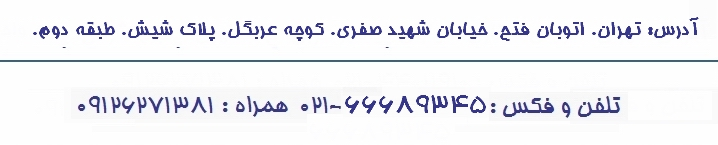©کليه حقوق مادي و معنوي اين سايت متعلق به این شرکت است. هر گونه کپي برداري از اطلاعات سايت بدون اطلاع مدير سايت ممنوع و ذکر تمامي مطالب با درج منبع بلامانع است.

منوی اصلی

لیست مقاله ها

لینک های ثابت

 واحد طراحی گروه مشاوره محصولات

اطلاع از به روز رسانی

 عضويت لغو عضويت

جستجو

 وب سایت